## Monday, May 23, 2016

### Estimating the evolutionary correlation between a multistate discrete character and a continuous trait under the threshold model: An ad hoc approah

Several people have expressed interest in fitting a correlational threshold model in which one or more of the threshold traits are multistate rather than binary. Such a trait would have to be ordered, in which subsequent states represent subsequent changes in liability across a series of two or more thresholds.

The main difficulty that this poses is that for multistate characters we must also estimate the relative positions of the thresholds. Unfortunately this is not straightforward. One reasonable option that I suggested in my 2014 paper is that the liabilities at the tips of the tree, the correlations between traits, and the threshold positions, could be sampled from their joint posterior distribution using MCMC. Unfortunately, no such approach has yet been implemented in R or other software.

What has been developed, though, is a function (`ancThresh`) in my package that can sample tip and node liabilites, and the positions of two or more thresholds, for a single trait using MCMC, primarily to the end of estimating ancestral character values under the model for that trait. One ad hoc, though creative, tactic that I have seen employed recently has been to first sample tip & node liabilities, and then use these liabilites in a standard phylogenetic correlation analysis. Though this seems quite reasonable, to my knowledge no one has yet explored the statistical properties of the method. This isn't hard to do, however, so I though I'd try to do it here.

``````library(phytools)
library(plotrix)
library(coda)
``````

Next, let's simulate a tree & some data. Here, I'll simulate no correlation (`r=0`) between the threshold trait and a second, continuous character:

``````## simulate tree
tree<-pbtree(n=26,scale=1,tip.label=LETTERS)
## simulated correlated data for two characters
r<-0
VCV<-matrix(c(1,r*sqrt(2),r*sqrt(2),2),2,2)
X<-sim.corrs(tree,VCV)
X[,1]<-X[,1]-mean(X[,1]) ## recenter character 1
## threshold trait 1
x<-sapply(X[,1],threshState,thresholds=setNames(c(0,1,Inf),
letters[1:3]))
x
``````
``````##   A   B   C   D   E   F   G   H   I   J   K   L   M   N   O   P   Q   R
## "c" "c" "c" "b" "a" "a" "a" "c" "a" "a" "a" "a" "a" "a" "a" "a" "a" "b"
##   S   T   U   V   W   X   Y   Z
## "b" "b" "b" "a" "a" "b" "b" "b"
``````
``````y<-X[,2]
y
``````
``````##           A           B           C           D           E           F
## -1.67106581 -1.38254877 -0.98622363 -0.66341721 -0.95826839 -0.33150388
##           G           H           I           J           K           L
##  1.02161990  0.04758419 -0.16356017 -0.05162870  0.34089327 -0.08999551
##           M           N           O           P           Q           R
##  0.06010288  0.61015394  0.27364931  0.58195450  0.48776407  0.63435560
##           S           T           U           V           W           X
## -0.13646326  0.77393761  0.71105093  0.65765914  1.23746620  1.00154033
##           Y           Z
##  1.09755746  1.32505288
``````

Now that is done, we can go ahead & sample tip & node liabilities, and the positions of the thresholds (actually, only one threshold, that between `b` & `c`, because the threshold between `a` and `b` is fixed) from their joint posterior distribution using Bayesian MCMC. This is for our single thresholded trait.

``````mcmc<-ancThresh(tree,x,ngen=200000,sequence=letters[1:3],
control=list(plot=FALSE,print=FALSE))
``````
``````## MCMC starting....
``````

Now that is complete, let's first compare the mean liabilities for the thresholded character to the underlying true liabilities, merely because they happen to be known in this case:

``````hpd<-function(x) HPDinterval(as.mcmc(x))
colors<-setNames(c("blue","red","green"),letters[1:3])
plotCI(X[,1],colMeans(mcmc\$liab[21:201,tree\$tip.label]),pch=19,
ui=apply(mcmc\$liab[21:201,tree\$tip.label],2,hpd)[2,],
li=apply(mcmc\$liab[21:201,tree\$tip.label],2,hpd)[1,],
xlab="true liabilities",ylab="estimated liabilities (& HPDs)",
col=colors[x],scol="black")
lines(par()\$usr[1:2],par()\$usr[1:2],lty="dashed",lwd=2,col="red")
abline(v=0,lty="dotted")
abline(h=0,lty="dotted")
``````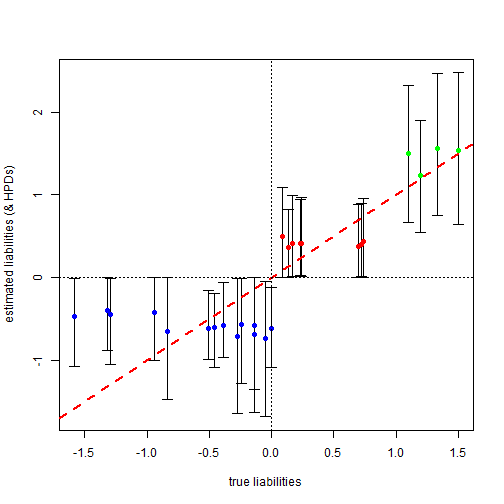Having done this we see that most of the true liability values, which we would not normally know of course, fall with the 95% HPD interval for the estimates.

Next, we can obtain a posterior distribution for the correlation between `x` & `y`. This is what we set out after in the first place, recall. Here what I'll do - instead of computing a single correlation from the posterior means - is compute a separate correlation from each post burn-in sample that I have from the posterior. Remember that I must take phylogeny into account when doing so!

``````foo<-function(x,y,tree)
cov2cor(phyl.vcv(cbind(x,y),vcv(tree),1)\$R)[1,2]
r.mcmc<-apply(mcmc\$liab[21:201,tree\$tip.label],1,foo,y=X[,2],tree=tree)
pd.r<-density(r.mcmc)
pd.r
``````
``````##
## Call:
##  density.default(x = r.mcmc)
##
## Data: r.mcmc (181 obs.); Bandwidth 'bw' = 0.04539
##
##        x                  y
##  Min.   :-0.57385   Min.   :0.0005589
##  1st Qu.:-0.31506   1st Qu.:0.0969140
##  Median :-0.05627   Median :0.6718680
##  Mean   :-0.05627   Mean   :0.9650849
##  3rd Qu.: 0.20251   3rd Qu.:1.9282196
##  Max.   : 0.46130   Max.   :2.5189292
``````
``````plot(pd.r,main="posterior density on the correlation",xlab="r")
abline(v=0,lty="dashed",lwd=2,col="red")
text(x=0,y=0.1,"generating value of r",pos=4)
``````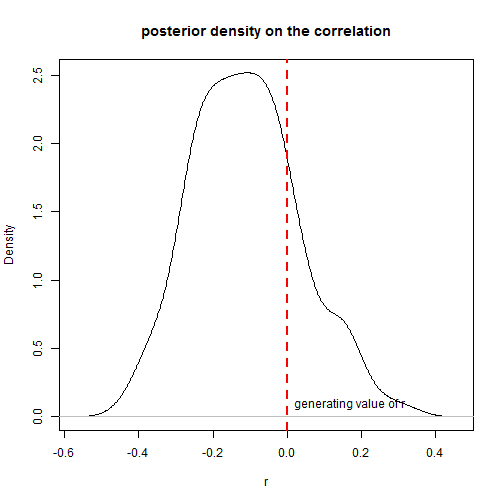``````ci<-HPDinterval(as.mcmc(r.mcmc))
ci
``````
``````##           lower     upper
## var1 -0.3705042 0.1758467
## attr(,"Probability")
##  0.9502762
``````

Of course, we would need to duplicate this a large number of times (but due the computationally instensive nature of the MCMC, we can start with 20) to get a general sense of the overall statistical properties of this approach. Let's try it:

``````nrep<-20
ci<-ci.cov<-matrix(NA,nrep,2)
R<-R.mean<-Cov<-Cov.mean<-vector()
mcmc<-vector(mode="list",length=nrep)
r<-0
VCV<-matrix(c(1,r*sqrt(2),r*sqrt(2),2),2,2)
corp<-function(x,y,tree)
cov2cor(phyl.vcv(cbind(x,y),vcv(tree),1)\$R)[1,2]
covp<-function(x,y,tree)
phyl.vcv(cbind(x,y),vcv(tree),1)\$R[1,2]
trees<-pbtree(n=26,scale=1,tip.label=LETTERS,nsim=nrep)
for(i in 1:nrep){
## simulation
X<-sim.corrs(trees[[i]],VCV)
X[,1]<-X[,1]-mean(X[,1])
x<-sapply(X[,1],threshState,thresholds=setNames(c(0,1,Inf),
letters[1:3]))
## MCMC
mcmc[[i]]<-ancThresh(trees[[i]],x,ngen=200000,sequence=letters[1:3],
control=list(plot=FALSE,print=FALSE))
r.mcmc<-apply(mcmc[[i]]\$liab[21:201,trees[[i]]\$tip.label],1,corp,
y=X[,2],tree=trees[[i]])
R[i]<-mean(r.mcmc)
ci[i,]<-HPDinterval(as.mcmc(r.mcmc))
## calculate a couple of other things here for comparison
R.mean[i]<-corp(colMeans(mcmc[[i]]\$liab[21:201,
trees[[i]]\$tip.label]),X[,2],trees[[i]])
cov.mcmc<-apply(mcmc[[i]]\$liab[21:201,trees[[i]]\$tip.label],1,covp,
y=X[,2],tree=trees[[i]])
Cov[i]<-mean(cov.mcmc)
ci.cov[i,]<-HPDinterval(as.mcmc(cov.mcmc))
Cov.mean<-covp(colMeans(mcmc[[i]]\$liab[21:201,
trees[[i]]\$tip.label]),X[,2],trees[[i]])
cat(paste("------------------------ Done replicate",i,"\n"))
}
``````
``````## MCMC starting....
``````
``````## ------------------------ Done replicate 1
``````
``````## MCMC starting....
``````
``````## ------------------------ Done replicate 2
``````
``````## MCMC starting....
``````
``````## ------------------------ Done replicate 3
``````
``````## MCMC starting....
``````
``````## ------------------------ Done replicate 4
``````
``````## MCMC starting....
``````
``````## ------------------------ Done replicate 5
``````
``````## MCMC starting....
``````
``````## ------------------------ Done replicate 6
``````
``````## MCMC starting....
``````
``````## ------------------------ Done replicate 7
``````
``````## MCMC starting....
``````
``````## ------------------------ Done replicate 8
``````
``````## MCMC starting....
``````
``````## ------------------------ Done replicate 9
``````
``````## MCMC starting....
``````
``````## ------------------------ Done replicate 10
``````
``````## MCMC starting....
``````
``````## ------------------------ Done replicate 11
``````
``````## MCMC starting....
``````
``````## ------------------------ Done replicate 12
``````
``````## MCMC starting....
``````
``````## ------------------------ Done replicate 13
``````
``````## MCMC starting....
``````
``````## ------------------------ Done replicate 14
``````
``````## MCMC starting....
``````
``````## ------------------------ Done replicate 15
``````
``````## MCMC starting....
``````
``````## ------------------------ Done replicate 16
``````
``````## MCMC starting....
``````
``````## ------------------------ Done replicate 17
``````
``````## MCMC starting....
``````
``````## ------------------------ Done replicate 18
``````
``````## MCMC starting....
``````
``````## ------------------------ Done replicate 19
``````
``````## MCMC starting....
``````
``````## ------------------------ Done replicate 20
``````

Having done that, we can then take a look at whether our mean correlation across replicates is more or less centered on the generating value of `r = 0`, and similarly what fraction of the 95% HPDs for the correlation include this generating value. We think it should be around 19/20 or 95%.

``````R
``````
``````##   -0.048566633 -0.005317359  0.146492590  0.119758935  0.041327768
##   -0.162323365  0.152224005 -0.143100612  0.043652399  0.107146396
##   0.327710842 -0.094010491  0.195777668 -0.001286759 -0.147711651
##   0.448247724 -0.094734121 -0.042752183 -0.269427398 -0.164817066
``````
``````mean(R)
``````
``````##  0.02041453
``````
``````ci
``````
``````##              [,1]         [,2]
##  [1,] -0.27823664  0.166015861
##  [2,] -0.31197924  0.324153803
##  [3,] -0.15500085  0.362459410
##  [4,] -0.06140886  0.359871967
##  [5,] -0.15890202  0.249944995
##  [6,] -0.39634111  0.166358895
##  [7,] -0.11452389  0.385336731
##  [8,] -0.32451440  0.049618284
##  [9,] -0.17915762  0.338527915
## [10,] -0.09885426  0.299924390
## [11,]  0.13334608  0.552577114
## [12,] -0.33714016  0.103557367
## [13,]  0.01927001  0.392790224
## [14,] -0.30864220  0.254575236
## [15,] -0.33681573  0.085616931
## [16,]  0.26108001  0.606684937
## [17,] -0.35611455  0.191008778
## [18,] -0.30745918  0.161326103
## [19,] -0.46888144 -0.006236106
## [20,] -0.46673940  0.050538976
``````
``````mean((ci[,1]<0)*(ci[,2]>0)==1)
``````
``````##  0.8
``````
``````## or
plotCI(x=R,y=1:nrep,ui=ci[,2],li=ci[,1],err="x",xlab="HPD(r)",
ylab="replicate",xlim=c(-1,1),pch=19)
abline(v=r,lty="dotted")
``````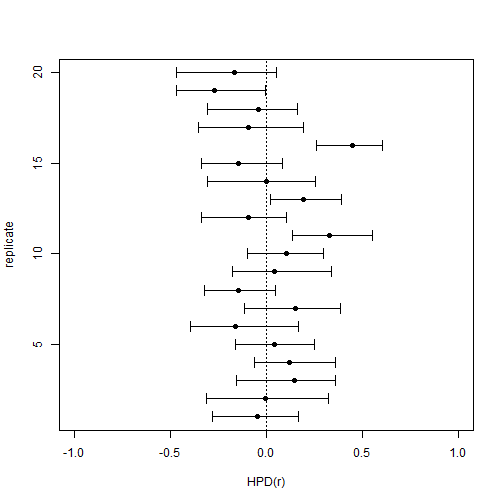Since we also computed the mean covariances & the correlations and covariance from the posterior mean liabilities for each run, let's compare these:

``````R.mean
``````
``````##   -0.059078345 -0.010050969  0.185637260  0.151141259  0.052384390
##   -0.217800815  0.230801440 -0.176791006  0.056176701  0.144713388
##   0.422623169 -0.128424671  0.262764952  0.002071499 -0.189752250
##   0.555325822 -0.137313778 -0.057192992 -0.344757074 -0.206130921
``````
``````mean(R.mean)
``````
``````##  0.02681735
``````
``````Cov
``````
``````##   -0.057641172 -0.008404547  0.166538292  0.208596920  0.039513951
##   -0.226406068  0.240540639 -0.208357788  0.068088427  0.142210715
##   0.478902164 -0.139405998  0.243998991  0.002606433 -0.217982679
##   0.678894959 -0.159555319 -0.071439376 -0.406861207 -0.226330470
``````
``````mean(Cov)
``````
``````##  0.02737534
``````
``````ci.cov
``````
``````##               [,1]       [,2]
##  [1,] -0.278687429  0.2653090
##  [2,] -0.420817922  0.3900768
##  [3,] -0.130056443  0.4623751
##  [4,] -0.150981029  0.5757184
##  [5,] -0.140800003  0.2304954
##  [6,] -0.601914477  0.1569817
##  [7,] -0.141190450  0.6656397
##  [8,] -0.512008852  0.0871602
##  [9,] -0.334170232  0.4869676
## [10,] -0.133863757  0.3928547
## [11,]  0.095436409  0.7851570
## [12,] -0.508154395  0.1572361
## [13,]  0.004246627  0.4784923
## [14,] -0.526237496  0.4009590
## [15,] -0.489581250  0.1587820
## [16,]  0.420823663  0.9959279
## [17,] -0.638616064  0.3115659
## [18,] -0.450950663  0.3122699
## [19,] -0.813975100 -0.1000418
## [20,] -0.564341396  0.1422885
``````
``````mean((ci.cov[,1]<0)*(ci.cov[,2]>0)==1)
``````
``````##  0.8
``````
``````## or
plotCI(x=Cov,y=1:nrep,ui=ci.cov[,2],li=ci.cov[,1],
err="x",xlab="HPD(cov)",ylab="replicate",
xlim=c(-2,2),pch=19)
abline(v=VCV[1,2],lty="dotted")
``````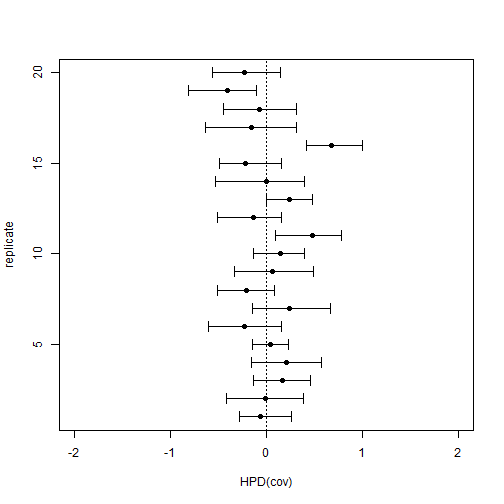Next, we can try with a correlation between `x` and `y`. For this, all I have to do is duplicate the above but change the correlation to some non-zero value. Here I have chosen `r = -0.7`, but this is totally arbitrary!

``````nrep<-20
ci<-matrix(NA,nrep,2)
R<-vector()
mcmc<-vector(mode="list",length=20)
r<--0.7
VCV<-matrix(c(1,r*sqrt(2),r*sqrt(2),2),2,2)
for(i in 1:nrep){
## simulation
X<-sim.corrs(trees[[i]],VCV)
X[,1]<-X[,1]-mean(X[,1])
x<-sapply(X[,1],threshState,thresholds=setNames(c(0,1,Inf),
letters[1:3]))
y<-X[,2]
## MCMC
mcmc[[i]]<-ancThresh(trees[[i]],x,ngen=200000,sequence=letters[1:3],
control=list(plot=FALSE,print=FALSE))
r.mcmc<-apply(mcmc[[i]]\$liab[21:201,trees[[i]]\$tip.label],1,corp,
y=X[,2],tree=trees[[i]])
R[i]<-mean(r.mcmc)
ci[i,]<-HPDinterval(as.mcmc(r.mcmc))
R.mean[i]<-corp(colMeans(mcmc[[i]]\$liab[21:201,
trees[[i]]\$tip.label]),X[,2],trees[[i]])
cov.mcmc<-apply(mcmc[[i]]\$liab[21:201,trees[[i]]\$tip.label],1,covp,
y=X[,2],tree=trees[[i]])
Cov[i]<-mean(cov.mcmc)
ci.cov[i,]<-HPDinterval(as.mcmc(cov.mcmc))
Cov.mean<-covp(colMeans(mcmc[[i]]\$liab[21:201,
trees[[i]]\$tip.label]),X[,2],trees[[i]])
cat(paste("------------------------ Done replicate",i,"\n"))
}
``````
``````## MCMC starting....
``````
``````## ------------------------ Done replicate 1
``````
``````## MCMC starting....
``````
``````## ------------------------ Done replicate 2
``````
``````## MCMC starting....
``````
``````## ------------------------ Done replicate 3
``````
``````## MCMC starting....
``````
``````## ------------------------ Done replicate 4
``````
``````## MCMC starting....
``````
``````## ------------------------ Done replicate 5
``````
``````## MCMC starting....
``````
``````## ------------------------ Done replicate 6
``````
``````## MCMC starting....
``````
``````## ------------------------ Done replicate 7
``````
``````## MCMC starting....
``````
``````## ------------------------ Done replicate 8
``````
``````## MCMC starting....
``````
``````## ------------------------ Done replicate 9
``````
``````## MCMC starting....
``````
``````## ------------------------ Done replicate 10
``````
``````## MCMC starting....
``````
``````## ------------------------ Done replicate 11
``````
``````## MCMC starting....
``````
``````## ------------------------ Done replicate 12
``````
``````## MCMC starting....
``````
``````## ------------------------ Done replicate 13
``````
``````## MCMC starting....
``````
``````## ------------------------ Done replicate 14
``````
``````## MCMC starting....
``````
``````## ------------------------ Done replicate 15
``````
``````## MCMC starting....
``````
``````## ------------------------ Done replicate 16
``````
``````## MCMC starting....
``````
``````## ------------------------ Done replicate 17
``````
``````## MCMC starting....
``````
``````## ------------------------ Done replicate 18
``````
``````## MCMC starting....
``````
``````## ------------------------ Done replicate 19
``````
``````## MCMC starting....
``````
``````## ------------------------ Done replicate 20
``````
``````R
``````
``````##   -0.4442685 -0.3329273 -0.2039185  0.1561997 -0.4330033 -0.5425256
##   -0.4794049 -0.4291188 -0.2694266 -0.3460377 -0.4258567 -0.4981087
##  -0.5232131 -0.5044816 -0.2361279 -0.3941872 -0.1844598 -0.4250402
##  -0.3504278 -0.5753514
``````
``````mean(R)
``````
``````##  -0.3720843
``````
``````ci
``````
``````##             [,1]         [,2]
##  [1,] -0.6461571 -0.188916059
##  [2,] -0.5258120 -0.095176138
##  [3,] -0.4636199  0.036734499
##  [4,] -0.1420690  0.408757105
##  [5,] -0.6239437 -0.198852437
##  [6,] -0.7197192 -0.295896109
##  [7,] -0.6642045 -0.274124877
##  [8,] -0.6582805 -0.207382961
##  [9,] -0.5618896  0.007048045
## [10,] -0.5650661 -0.125165941
## [11,] -0.5975407 -0.224868117
## [12,] -0.7031133 -0.248217990
## [13,] -0.6752238 -0.357285876
## [14,] -0.6738202 -0.325900549
## [15,] -0.5726131 -0.015487077
## [16,] -0.5936548 -0.150543085
## [17,] -0.4405038  0.037160021
## [18,] -0.6518851 -0.209177296
## [19,] -0.6380389 -0.039961077
## [20,] -0.7346678 -0.406110586
``````
``````mean((ci[,1]<r)*(ci[,2]>r)==1)
``````
``````##  0.15
``````
``````## or
plotCI(x=R,y=1:nrep,ui=ci[,2],li=ci[,1],err="x",xlab="HPD(r)",
ylab="replicate",xlim=c(-1,1),pch=19)
abline(v=r,lty="dotted")
``````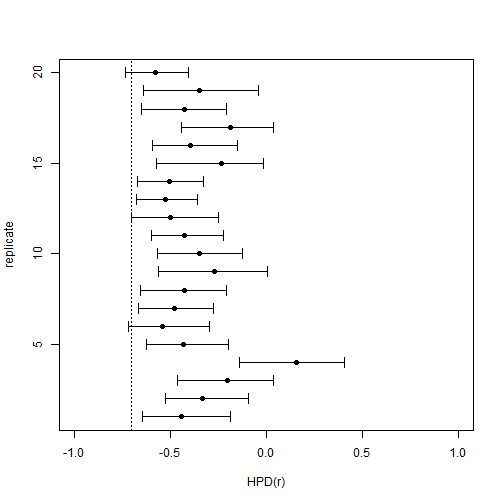``````R.mean
``````
``````##   -0.5845377 -0.4564454 -0.2887876  0.2126492 -0.5455727 -0.7034432
##   -0.6610401 -0.5528324 -0.3826757 -0.4268218 -0.5151527 -0.6099373
##  -0.6110249 -0.6154331 -0.3824219 -0.5115940 -0.2494795 -0.5614771
##  -0.5375527 -0.7291751
``````
``````mean(R.mean)
``````
``````##  -0.4856378
``````
``````Cov
``````
``````##   -0.5333285 -0.5084913 -0.2576849  0.1644342 -0.6660364 -0.9119713
##   -0.8007782 -0.6881301 -0.4577610 -0.5032624 -0.5714295 -0.7532690
##  -0.9704486 -0.7441925 -0.3652020 -0.5726135 -0.2391976 -0.6613127
##  -0.4382249 -0.7324093
``````
``````mean(Cov)
``````
``````##  -0.5605655
``````
``````ci.cov
``````
``````##             [,1]        [,2]
##  [1,] -0.8027139 -0.23520232
##  [2,] -0.8881685 -0.13503644
##  [3,] -0.5809815  0.06139351
##  [4,] -0.1515432  0.44017473
##  [5,] -1.0456683 -0.31672684
##  [6,] -1.3254044 -0.46230969
##  [7,] -1.1891047 -0.39266423
##  [8,] -1.0838651 -0.30619133
##  [9,] -0.9962171  0.01140984
## [10,] -0.8123446 -0.10359772
## [11,] -0.8514475 -0.30431646
## [12,] -1.1551143 -0.38880466
## [13,] -1.3674045 -0.55207820
## [14,] -1.0904980 -0.40602170
## [15,] -0.8448122  0.01013859
## [16,] -0.8779924 -0.19469199
## [17,] -0.5146207  0.10636017
## [18,] -1.0223800 -0.25280889
## [19,] -0.7684456  0.06299185
## [20,] -0.9752561 -0.43084719
``````
``````mean((ci.cov[,1]<VCV[1,2])*(ci.cov[,2]>VCV[1,2])==1)
``````
``````##  0.45
``````
``````## or
plotCI(x=Cov,y=1:nrep,ui=ci.cov[,2],li=ci.cov[,1],
err="x",xlab="HPD(cov)",ylab="replicate",
xlim=c(-2,2),pch=19)
abline(v=VCV[1,2],lty="dotted")
``````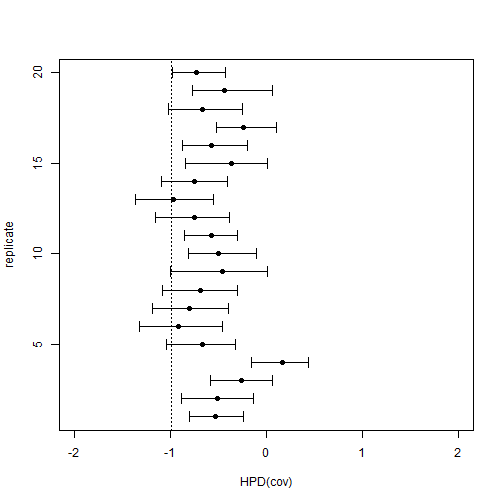This is a very small experiment, but it at least suggests that this tactic probably does not result in vastly inflated type I error under the null hypothesis. Furthermore, it may not be too bad at estimating the evolutionary correlation - although better with the mean from the posterior (than the mean correlation computed from each sample), and better still with the covariance. (This latter result is not too surprising as we have thrown out most of the information about the evolutionary correlation by thresholding our character trait, so this will probably bias our correlation towards zero.)

One thing perhaps worth noting is that I have done nothing here to assess genuine convergence to the posterior distribution of my Bayesian MCMC runs. A proper analysis of the statistical properties of the method would necessitate this. Furthermore, a failure to converge could result in HPDs that are both too broad or too narrow, as well as systematic bias in the mean correlation from the posterior sample.

Perhaps more on this later….

#### 1 comment:

1.Dear Dr. Revell,
I am conducting a comparative analysis using threshold models. I have a problem concerning the convergence. A failure to converge the posterior distribution using a specific ordering character could mean that such an ordering is incorrect? When I use a specific ordering, I get convergence. Conversely, other orderings do not converge even using big samples.
Is there a way to adjust the control argument in the ancThresh function?
I am looking forward to rearing from you and thank you in advance for your time and consideration regarding this matter.​

Note: due to the very large amount of spam, all comments are now automatically submitted for moderation.